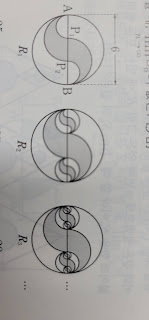# Sage code for making fractal picturesAnonymous

I am a person who makes math problems by coding in Korea.

I need to write a question (about geometric sequences) using pictures, but I don't know how to make them.I know it's difficult to tell all of them, but I would appreciate pointers to related sources or examples.

edit retag close merge delete

Edited the question to turn the urls into image links. Not sure how to rotate by a quarter turn. Maybe the original poster can upload rotated pictures somewhere.

Sort by » oldest newest most votedThe following code may help to get one of the pictures:

def figure(c,r,z):
fig = circle((c,0), r, facecolor="white", edgecolor="black", fill=True, zorder=z)
fig += disk((c+r/3,0), 2*r/3, (0,pi), color="lightgray", zorder=z+1)
fig += arc((c+r/3,0), 2*r/3, sector=(0,pi), color="black", zorder=z+1)
fig += disk((c-r/3,0), 2*r/3, (pi,2*pi), color="lightgray", zorder=z+1)
fig += arc((c-r/3,0), 2*r/3, sector=(pi,2*pi), color="black", zorder=z+1)
fig += disk((c+2*r/3,0), r/3, (0,pi), color="white", zorder=z+2)
fig += arc((c+2*r/3,0), r/3, sector=(0,pi), color="black", zorder=z+2)
fig += disk((c-2*r/3,0), r/3, (pi,2*pi), color="white", zorder=z+2)
fig += arc((c-2*r/3,0), r/3, sector=(pi,2*pi), color="black", zorder=z+2)
fig += line([(c-r,0),(c+r,0)], color="black", zorder=z+2)
return fig
centers = [[2/3], [4/9, 8/9], [10/27,14/27,22/27,26/27]]
zs = [5, 10, 15]
r = 3
R = figure(0,r,0)
show(R, axes=False)
for i in [0,1,2]:
for c in centers[i]:
show(R, axes=False)


See this SageMath cell for the output.

more